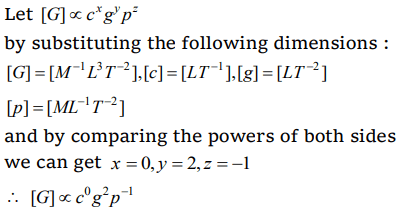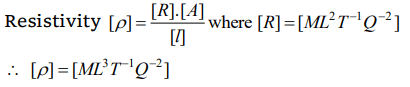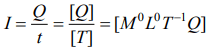## Units and Measurement Questions and Answers Part-20

1. The dimensions of inter atomic force constant are
a) $MT^{-2}$
b) $MLT^{-1}$
c) $MLT^{-2}$
d) $ML^{-1} T^{-1}$

Explanation:2. If the speed of light (c) , acceleration due to gravity (g) and pressure (p) are taken as the fundamental quantities, then the dimension of gravitational constant is
a) $c^{2} g^{0} p^{-2}$
b) $c^{0} g^{2} p^{-1}$
c) $c g^{3} p^{-2}$
d) $c^{-1} g^{0} p^{-1}$

Explanation:3. If the time period $\left(T\right)$ of vibration of a liquid dropdepends on surface tension $\left(S\right)$ , radius $\left(r\right)$ of the drop and density $\left(\rho\right)$ of the liquid, then the expression of T is
a) $T=k\sqrt{\rho r^{3}\diagup S}$
b) $T=k\sqrt{\rho^{1\diagup 2} r^{3}\diagup S}$
c) $T=k\sqrt{\rho r^{3}\diagup S^{1\diagup 2}}$
d) None of these

Explanation:4. $ML^{3}T^{-1}Q^{-2}$   is dimension of
a) Resistivity
b) Conductivity
c) Resistance
d) None of these

Explanation:5. Dimension of electric current is
a) $M^{0}L^{0}T^{-1}Q$
b) $ML^{2}T^{-1}Q$
c) $M^{2}LT^{-1}Q$
d) $M^{2}L^{2}T^{-1}Q$

Explanation:6. The fundamental physical quantities that have same dimensions in the dimensional formulae of torque and angular momentum are
a) Mass, time
b) Time, length
c) Mass, length
d) Time, mole

Explanation: Torque = [ML2T-2] , Angular momentum = [ML2T-1]. So mass and length have the same dimensions

7. If pressure P , velocity V and time T are taken as fundamental physical quantities, the dimensional formula of force is
a) $PV^{2}T^{2}$
b) $P^{-1}V^{2}T^{-2}$
c) $PVT^{2}$
d) $P^{-1}VT^{2}$

Explanation:8. The physical quantity which has dimensional formula as that of $\frac{Energy}{Mass\times Length}$   is
a) Force
b) Power
c) Pressure
d) Acceleration

Explanation:9. If energy $\left(E\right)$ , velocity $\left(v\right)$ and force $\left(F\right)$ be taken as fundamental quantity, then what are the dimensions of mass
a) $Ev^{2}$
b) $Ev^{-2}$
c) $Fv^{-1}$
d) $Fv^{-2}$a) $ML^{2}T^{-2}$
b) $ML^{2}T^{-3}$
c) $ML^{2}T^{-1}$
d) $MLT^{-2}$
Explanation: $ML^{2}T^{-3}$